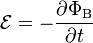# Lenz's law facts for kids

Kids Encyclopedia Facts

Lenz's law is a common way to understand how electromagnetic circuits obey Newton's third law and the conservation of energy. Lenz's law is named after Heinrich Lenz, and it says:

An induced electromotive force (emf) always gives rise to a current whose magnetic field opposes the original change in magnetic flux.$\mathcal{E}=-\frac{\partial \Phi_\mathrm{B}}{\partial t}$,

which indicates that the induced emf (ℰ) and the change in magnetic flux (∂ΦB) have opposite signs.

The induced emf and the resulting induced current are counterclockwise when B is directed out from the page and the area of the circuit is decreasing. The flux through this circuit is decreasing in the outward direction. Now the induced current I produces its own magnetic field, and we may use the right-hand grip rule to compute the direction of this field. The result is that the magnetic field due to the induced current is also directed outward within the circuit. It is as though nature, through this induced field, tried to compensate for the reduction in the flux due to the applied field B. This turns out experimentally to be a general rule, so that we may say that
The direction of the induced emf is always such as to result in opposition to the change producing it.
That is Lenz's law.

As another example of the application of Lenz's law, consider a coil of wire to which a battery is suddenly connected. Suppose that the battery starts a current flowing clockwise, as viewed by the observer. This current will give rise to a magnetic field whose lines will thread the coil and circle back outside it. Thus, as the current due to the battery builds up, there is a changing magnetic flux through the coil and this must result in an induced emf in the coil. What is the direction of this induced emf? Lenz's law tells us immediately that it must be counterclockwise, so as to oppose the building up of the current. Similarly, when the current in a circuit is broken, the induced emf seeks to keep the current from dying out, and this accounts for the sparking observed when switches are opened slowly. The induced emf in a circuit whose current is changing is called the back emf, since it always opposes the alteration in the current. It arises from change in the current's own magnetic field, an effect referred to as self-inductance.

Were Lenz's law not true, an increase in current in a coil would result in an emf that aided the applied battery, thus increasing the current further, inducing more emf and further increases in the current, ad infinitum. This would be an unstable situation and one in which the conservation of energy principle would be disobeyed.
This sort of reasoning may be extended to other situations in which a system in equilibrium is displaced and the principle arrived at is the following.

When a system in equilibrium is disturbed, the equilibrium is displaced in the direction which tends to undo the effects of the disturbance.
This generalization of Lenz's law is called Le Chatelier's prinicple.Lenz's law Facts for Kids. Kiddle Encyclopedia.